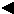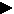Tenacity Conversion Factors R.S. Krowicki and R.J. Kohel ABSTRACT The following information is presented to aid in the interpretation, use and conversion of measures of cotton fiber tenacity. Definitions grex -- a unit of linear density based on the weight in grams (g) of 10 kilometer (km) of fiber. tex -- a unit of linear density based on the weight in grams (g) of a kilometer (km) of fiber. tenacity -- tensile stress or the force to break the cohesive forces for a unit cross-sectional area expressed in terms of force per unit linear density. Measurements and Relationships Stelometer force is expressed in terms of kilograms (kg). Linear density is determined from the weight of fiber in milligrams (mg) divided by the length of the fibers weighed (1.5 centimeters (cm) for T(1) when the linear density in terms of grex was used and 15 millimeters (mm) for T(1) when the linear density in terms of tex is used). Tenacity is determined by dividing the breaking force by the linear density.[Previous] [Next]